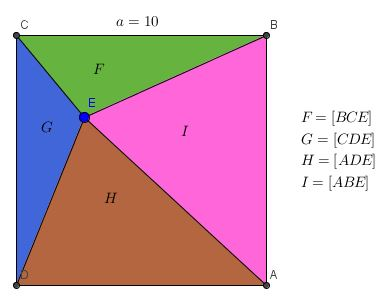# Are There Even Enough Information?

Geometry Level 2Above shows a square $ABCD$ with side length $a=10$. There is a point $E$ inside that square, that forms four triangles $F$,$G$,$H$ and $I$ with the corners of the square $A$,$B$,$C$ and $D$, respectively as shown in the picture above.

Find the sum of areas, $F+H$.

If you think there are not enough information to solve, type 3141 as your answer.

×